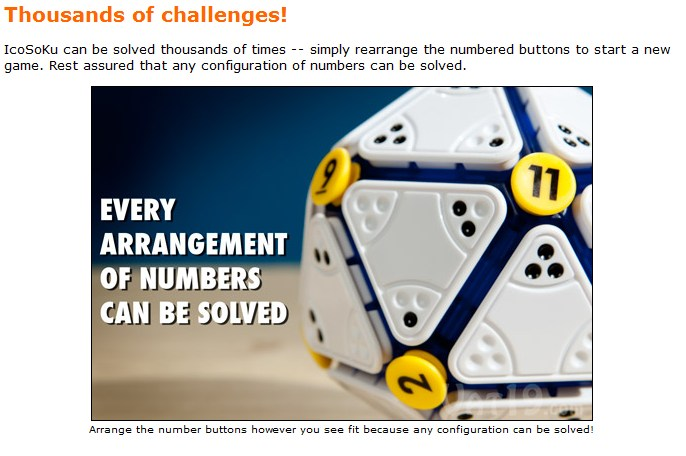# IcoSoKu

From the brilliant(?) minds at vat19.com, I present to you, the IcoSoKu:Just thousands, that’s it?

In my list of mathematical strengths probability does not appear, but there are: 12 unique numbers (vertices of an icosahedron), so 12! = 479,001,600 ways to arrange them. But because of the symmetry involved in a icosahedron, there are 12 ways to arrange a single icosahedron. So 12! / 12 = 11! = 39,916,800 ways to arrange the vertices. If each setup of vertices only has one solution, then that’s it, but I’m not sure if each solution is unique. If each tile is unique, then there are 20! ways to arrange the tiles, but I don’t think that comes into play for the number of challenges.

Am I missing anything? Is this interesting? Keep delving?

Anyway, interesting use of Platonic solids. BTW, bought it and it’s in the mail.

## 7 thoughts on “IcoSoKu”

1.pat ballew says:

Dan,
Not sure if you meant them to be different, but you counted chiral images (right and left handed) as different in your method. For example looking down at the ONE vertex, you could have one with 2,3,4,5,6 around its adjacent vertices in either clockwise or anti-clockwise order and every other part of the two would be similarly 3-space mirror reflections of the other.

also I notice that there are 1,2,3, or zero black dots on some of the triangular faces so the vertices are differentiable and you could be back to 12factorial.

1.Dan says:

Great point! You would have thought that I should have caught the chiral issue because I just finished the latest Radiolab, Symmetry. I wonder if the chiral issue doesn’t apply because although there may be two identical arrangements (“left-handed” and “right-handed”), the triangle pieces themselves are not symmetrical in the same way, so the left and right hand arrangements may have different solutions. Hmmmm.

2.Dan Bourque says:

Hi all,

Being a computer nerd, I decided to code an algorithm to solve the minimal pieces for any number of pegs. Is anyone else interested in this? If enough people are interested, I’ll deploy my IcoSoKu Solver as a web application. I’m also hoping that, for each peg placed on the sphere, I could quickly calculate and display the number of possible solutions. As you’d drag-n-drop more pegs onto the sphere, that number would keep shrinking. Whenever I determine that only one tile can fit in a particular location, I’d reveal it.

Please let me know if you’re interested in such a web app. (This is just a weekends-and-evenings project for me, so I have no idea how long it will take me).

3.Raven McIntyre says:

Actually, while your idea of starting with 12! is accurate, you need to divide by 60, not 12 to achieve the true final number. With a defined configuration, you can have twelve posiotions, but with a given number in a position, you can have five rotational positions around it. Therefore, 12•5=60 positions for any configuration, yielding just under eight million solutions.

For the triangles, you would start LIKE 20!, but actually, because the tile has three positions, the initial tile would have 20•3=60 options, the second would be 57, the third 54, and so on, yielding a number that is approximately 8.5×10^27. Though, as I think about it, that would need to be divided by 60 as well to weed out most of the duplicates, and the vast majority of them will not be viable solutions.

Anyhow, I’m looking for strategies to solve the Icosoku, as the last two or three pieces routinely thwart me.

4.Craig says:

I would think the best algorithm to solve this would be to randomly pick to triangles and switch them. Then orient them in positions which minimize the error between the vertices (the twelve numbers) and the sum of the dots on the triangles corresponding to a vertex. If the error is improved, then keep the switch. If not, then switch back. Eventually, you should reach the solution. How long does it take? I don’t know. But my guess is that it is quick. This general idea is a Markov Chain Monte Carlo algorithm. http://en.wikipedia.org/wiki/Markov_chain_Monte_Carlo See also http://en.wikipedia.org/wiki/Markov_chain_Monte_Carlo

1.Craig says:
1.Craig says:

Correction: If the error is not improved, flip a coin. (The odd of heads should be specially calculated as in the Diaconis article.)